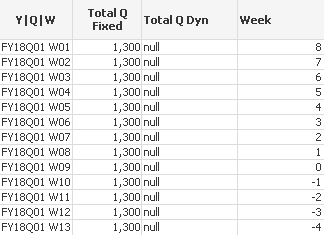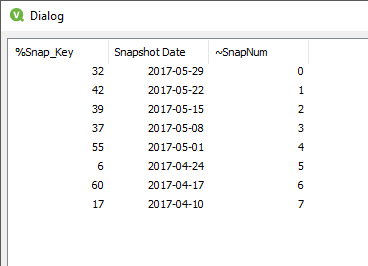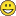Announcements
You can succeed best and quickest by helping others to succeed. Join the conversation.
cancel
Showing results for
Did you mean:Not applicable

## Variable in Set Analysis

Hi,

i use variable for special week number in Set Analysis, but is not functioning as intended. Variable calculate week number as follows:

next week => -1

actual week => 0

previous week => 1

and so on...

Result is integer number.

When i use this variable in table as expression, it works fine. (column Week)

When i use it in Set Analysis result is null. (Total Q Dyn)

When i use formula with set analysis with fixed number instead of variable, it works fine. (Total Q Fixed)

Here is my whole calculation:

1. LET vToday = Date(Today(),'YYYYMMDD');

2. vCurrentWeek = Only( {1<[DateKey]={'\$(vToday)'}>} QWNum) - take current week from Calendar

3. vWeekShift = (QWNum - \$(=\$(vCurrentWeek)))*(-1) - calculate week number in tableand set analysis:

4. SUM({\$<[~SName] = {'EBR'}, QWNum =, RepYQW =, [~SnapNum] = {\$(vWeekShift)}>} TOTAL (Quantity))

Snapshot Dimension:I don not know why, that specific variable is not usable in set analysis.

Result should be: for actual week it should count from snapshot with [~SnapNum] = 0, for previous week (1) should count from snapshot with [~SnapNum] = 1 and so on. No calendar filter should apply on this calculation.

Can you help me?

Thanks.

8 RepliesCreator

You forget the ' symbol

try this: '\$(vWeekShift)'Not applicable
Author

I already try that, no change.... And it is a number so a don not have to use ' symbol, i supposed.Anonymous
Not applicable
Author

Hello Jozef,

Try this in set analysis:

[~SnapNum] = {'=\$(vWeekShift)'}

Regards

Jacek.Partner - Champion III

vWeekShift is not defined with an aggregation function (like Max()/Min()...), so it will have no unique value in the set expression. Also the set expression and variable expansion happen before the chart is evaluated, so you cannot execute these on a row by row basis as I think you require.

Logic will get you from a to b. Imagination will take you everywhere. - A EinsteinNot applicable
Author

I try some combinations, how to put that variable into set analysis, but with no success. I think there is something with variable interpretation in set analysis. 😕 I try your idea, but the same result.Not applicable
Author

uff... ok this is a problem, so i have to find out, how to change the variable...Partner - Champion III

You know that the data set specified by your set analysis expression is calculated before the table object is calculated? And based on all data that is currently active (including selections and so on) or on the total data set if you used {1} inside your set analysis expression?

If you take that into account, you should be able to copy your variable \$(vWeekShift) equally well outside of every other object, like in a text box. Does it return a value then? Knowing that to calculate vWeekShift, you use field QWNum as a first operand and without aggregation function on your entire data set, I wouldn't think so.

BTW you can easily find the troublesome part in a complex variable-based expressions by pulling it apart, putting the pieces in text boxes and observing what happens. In your case, the calculation of vCurrentWeek works like a charm in a text box, but vWeekShift doesn't...Creator